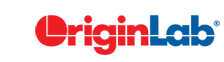# 3.121 FAQ-695 What is the difference between Integrate, integratexy and Integ1?

Last Update: 4/28/2015

You may notice that Origin has three integrate functions, integrate, integratexy and integ1.

integratexy integ1. integrate
Brief Description Takes a pair of X and Y data and calculates the cumulative values of integrated area under the curve. Missing values will be ignored. Takes a pair of X and Y data and calculates the mathematical area or absolute area under the curve. Takes a set of Y data and calculates the cumulative values of integrated area under the curve. Row numbers other than the associated X are used as X values of the curve. Missing values will be ignored.
Options it provides Calculate the mathematical area
• Calculate the mathematical area or absolute area
• Can create a baseline by straightly connecting end points.
• Multiple other values can output to the Result Log, including the values/indece of the beginning and ending X, maximum height and the corresponding X, and HWFH.
Calculate the mathematical areas
Script example
newbook;
col(a)={2:2:100};
col(b)=
nlf_gauss(col(a),-20,50,20,2000);
col(c)=
IntegrateXY(col(a), col(b));
newbook;
col(a)={2:2:100};
col(b)=
nlf_gauss(col(a),-20,50,20,2000);
integ1 iy:=col(b) type:=abs;
newbook;
col(a)={2:2:100};
col(b)=
nlf_gauss(col(a),-20,50,20,2000);
col(c)=Integrate(col(b));

Keywords:Integrate

Minimum Origin Version Required: 9.0